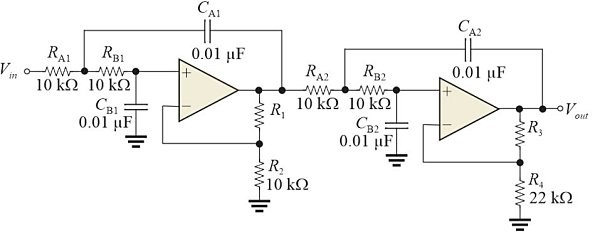Top Posters
Since Sunday

## QuestionFigure 1 Stage 1: DF = 1.848 and R1/R2 = 0.152; stage 2: DF = 0.765 and R3/R4 =1.235.

Refer to Figure 1 with values as given. R3 should have a value of

• 1.5 kΩ

• 1.2 kΩ

• 1.8 kΩ

• none of the above